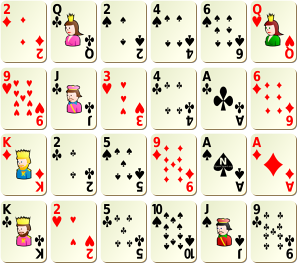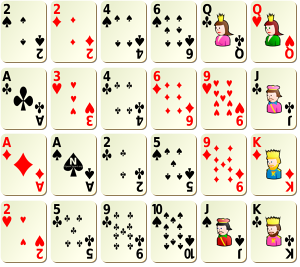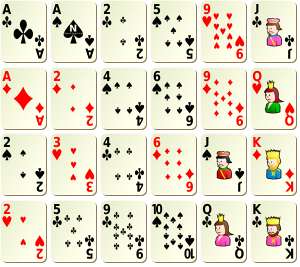# INF7100, “rappels” de maths

En parallèle des trois parties de mon intervention sur la science des données, dans le cadre du cours INF7100, j’ai mis en ligne une série des capsules de “rappels” de maths.

• 401: Concepts généraux (logarithme, exponentielle, dérivée, intégrale, approximation) pdf video (38:57)
• 411: Optimisation (condition du premier ordre, descente de gradient, Lagrangien) pdf video (31:33)
• 412: Ensembles (intersection, unions, fusion de bases, convexité, centroïdes) pdf video (10:13)
• 421: Vecteurs et Matrices (orthogonalité, distance, norme, tenseurs) pdf video (43:20)
• 422: Algèbre Linéaire (déterminant, valeurs propres, vecteurs propres, formes quadratiques, approximations) pdf
• 423: Coordonnées (données circulaires, coordonnées polaires, projection, spatial) pdf
• 431: Loi Gaussienne (loi normale, cas multivariée, limite et approximation, loi des grands nombres) pdf video (17:37)
• 432: Lois Puissance (changement d’échelle, loi puissance, Zipf, Benford) pdf
• 433: Loi de Durée (loi géométrique, loi exponentielle, loi de Poisson, loi des petits nombres) pdf
• 434: Modèle de Markov (données séquentielles) pdf

# Sorting rows and colums in a matrix (with some music, and some magic)

This morning, I was working on some paper on inequality measures, and for computational reasons, I had to sort elements in a matrix. To make it simple, I had a rectangular matrix, like the one below,

```> set.seed(1)
> u=sample(1:(nc*nl))
> (M1=matrix(u,nl,nc))
[,1] [,2] [,3] [,4] [,5] [,6]
[1,]    7    5   11   23    6   17
[2,]    9   18    1   21   24   15
[3,]   13   19    3    8   22    2
[4,]   20   12   14   16    4   10```

I had to sort elements in this matrix, by row.

```> (M2=t(apply(M1,1,sort)))
[,1] [,2] [,3] [,4] [,5] [,6]
[1,]    5    6    7   11   17   23
[2,]    1    9   15   18   21   24
[3,]    2    3    8   13   19   22
[4,]    4   10   12   14   16   20```

Nice, elements are sorted by row. But for symmetric reasons, I also wanted to sort them by column. So from this sorted matrix, I decided to sort elements by column,

```> (M3=apply(M2,2,sort))
[,1] [,2] [,3] [,4] [,5] [,6]
[1,]    1    3    7   11   16   20
[2,]    2    6    8   13   17   22
[3,]    4    9   12   14   19   23
[4,]    5   10   15   18   21   24```

Nice, elements are sorted by column now. Wait… elements are also sorted by row. How comes ? Is it some coincidence ? Actually, no, you can try…

```> library(scatterplot3d)
> nc=6; nl=5
> set.seed(1)
> u=sample(1:(nc*nl))
> (M1=matrix(u,nl,nc))
[,1] [,2] [,3] [,4] [,5] [,6]
[1,]    8   23    5   30   10   15
[2,]   11   27    4   29   21   28
[3,]   17   16   13   24   26   12
[4,]   25   14    7   20    1    3
[5,]    6    2   18    9   22   19
> M2=t(apply(M1,1,sort))
> M3=apply(M2,2,sort)```
```> M3
[,1] [,2] [,3] [,4] [,5] [,6]
[1,]    1    3    7   14   19   22
[2,]    2    6    9   15   20   25
[3,]    4    8   10   17   23   26
[4,]    5   11   16   18   24   29
[5,]   12   13   21   27   28   30```

or use the  following function is two random matrices are not enough,

```> doublesort=function(seed=2,nl=4,nc=6){
+ set.seed(seed)
+ u=sample(1:(nc*nl))
+ (M1=matrix(u,nl,nc))
+ (M2=t(apply(M1,1,sort)))
+ return(apply(M2,2,sort))
+ }```

Please, feel free to play with this function. Because this will always be the case. Of course, this is not a new result. Actually, it is mentioned in More Mathematical Morsels by Ross Honsberger, related to some story on marching band. The idea is simple: consider a marching band, a rectangular one. Here are my players

```> library(scatterplot3d)
> scatterplot3d(rep(1:nl,nc),rep(1:nc,each=nl), as.vector(M1),
+ col.axis="blue",angle=40,
+ col.grid="lightblue", main="", xlab="", ylab="", zlab="",
+ pch=21, box=FALSE, cex.symbols=1,type="h",color="red",axis=FALSE)```Quite messy, isn’t it ? At least, this is what the leader of the band though, since some tall players were hiding shorter ones. So, he brought the shorter ones forward, and moved the taller ones in the back. But still on the same line,

```> m=scatterplot3d(rep(1:nl,nc),rep(1:nc,each=nl), as.vector(M2),
> col.axis="blue",angle=40,
+ col.grid="lightblue", main="", xlab="", ylab="", zlab="",
+ pch=21, box=FALSE, cex.symbols=1,type="h",color="red",axis=FALSE)```From the leader’s perspective, everything was fine,

```> M=M2
> for(i in 1:nl){
+ for(j in 1:(nc-1)){
+ pts=m\$xyz.convert(x=c(i,i),y=c(j,j+1),z=c(M[i,j],M[i,j+1]))
+ segments(pts\$x,pts\$y,pts\$x,pts\$y)
+ }}```But someone in the public (on the right of this graph) did not have the same perspective.

```> for(j in 1:nc){
+ for(i in 1:(nl-1)){
+ pts=m\$xyz.convert(x=c(i,i+1),y=c(j,j),z=c(M[i,j],M[i+1,j]))
+ segments(pts\$x,pts\$y,pts\$x,pts\$y)
+ }}```So the person in the audience ask – one more time – players to move, but this time, to match with his perspective. Since I consider someone on the right, some minor adjustments should be made here

```> sortrev=function(x) sort(x,decreasing=TRUE)
> M3b=apply(M2,2,sortrev)```

This time, it is much bettter,

```> m=scatterplot3d(rep(1:nl,nc),rep(1:nc,each=nl), as.vector(M3b),
+ col.axis="blue",angle=40,
+ col.grid="lightblue", main="", xlab="", ylab="", zlab="",
+ pch=21, box=FALSE, cex.symbols=1,type="h",color="red",axis=FALSE)```And not only from the public’ perspective,

```> M=M3b
> for(j in 1:nc){
+ for(i in 1:(nl-1)){
+ pts=m\$xyz.convert(x=c(i,i+1),y=c(j,j),z=c(M[i,j],M[i+1,j]))
+ segments(pts\$x,pts\$y,pts\$x,pts\$y)
+ }}```but also for the leader of the marching bandNice, isn’t it ? And why is this property always valid ? Actually, it comes from the pigeonhole theorem (one more time), a nice explanation can be found in The Power of the Pigeonhole by Martin Gardner (a pdf version can also be found on http://www.ualberta.ca/~sgraves/..). As mentioned at the end of the paper, there is also an interpretation of that result that can be related to some magic trick, discussing – in picture – a few month ago on http://www.futilitycloset.com/… : deal cards into any rectangular array:Then put each row into numerical order:Now put each column into numerical order:That last step hasn’t disturbed the preceding one: rows are still in order. And that’s a direct result from  pigeonhole theorem. That’s awesome, isn’t it ?